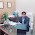1.             A man walks at certain place and rides back in 10 hours. He could ride both ways in 8 hours. The time taken by him to walk both ways?
A.       10 hr

B.       12 hr
C.        14 hr
D.       16 hr

2.            An inspector is 228 meter behind the thief. The inspector runs 42 meters and the thief runs 30 meters in a minute. In what time will the inspector catch the thief?
A.       19 minutes
B.       18 minutes
C.        20 minutes
D.       21 minutes

3.            A girl goes to her school from her house at a speed of 6km/hr and returns at a speed of 4 km/hr. If she takes 10 hours in going and coming back, the distance between her school and house is
A.       12km
B.       16km
C.        20km
D.       24km

4.            A walks with a speed of 6 km/hr and after 5 hr of his start, B starts running towards A at a speed of 8 km/hr. At what distance from start will B catch A.
A.       100 Km
B.       110 Km
C.        120Km
D.       140 Km

5.            A man purchases some apples at the rate of 3 for Rs 4 and same quantity at 4 for Rs 7. If he sells all the apples at the rate of 5 for Rs 9, find his gain or loss percent?
A.       17% loss
B.       15% loss
C.        17% gain
D.       15% gain

6.            A shopkeeper buys 60 cycles and marks them at 20% above the cost price. He allows a discount of 10% on the marked price for cash sale and 5% discount for credit sales. If three-fourth of the cycles are sold at cash and remaining for credit, the total profit be Rs. 11400. What is the cost price of a cycle?
A.       1000
B.       1500
C.        2000
D.       4000

7.            A defective chair costing rupees 2000 is being sold at 10% loss. If the price is further reduced by 8 percent. Find its approximate selling price?
A.       1556
B.       1656
C.        1756
D.       1956

8.           The percent profit when an article is sold for rupees 400 is twice than when the same article is sold for 250. The cost price of the article is-
A.       100
B.       150
C.        180
D.       200

9.            A reduction of 20% percent in the price of rice enables a housewife to buy 5 kg more for rupees 1200. The reduced price per kg of rice
A.       36
B.       45
C.        48
D.       60

10.        The population of a village has increased annually at the rate of 20%. If at the end of 3 years it is 21600, the population in the beginning of the first year?
A.       10000
B.       12500
C.        17500
D.       15000

1.I want to know how to solve the problem,
Sum is, , the radius circle whose centre is ( 3, 2 ) and passes through ( - 5, 6 ).
Let me know.

1.2.